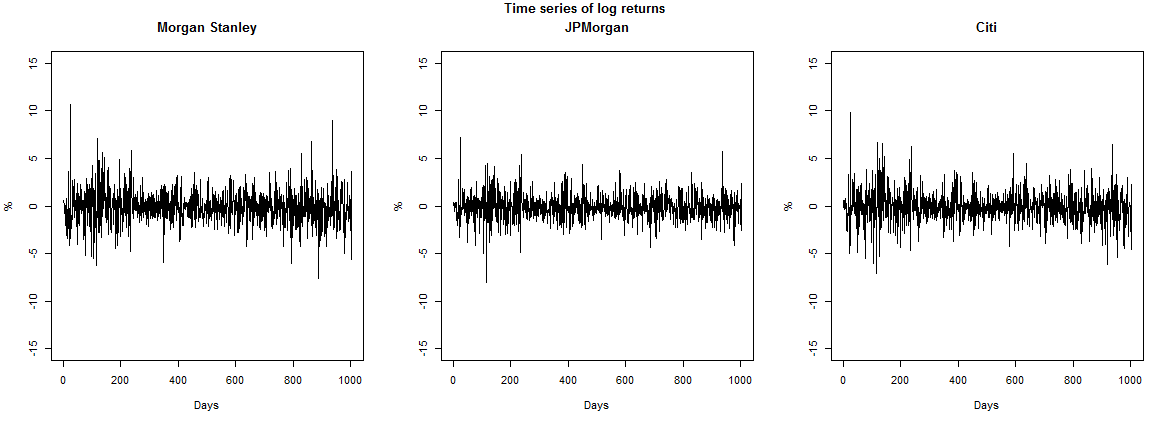# Multivariate stable distributions in finance

My name is Szabolcs Majoros. I am from Hungary, studying Actuarial and Financial Mathematics (MSc) at Eötvös Loránd University and I wrote a paper about parameter estimation of multivariate stable distributions for TDK (Scientific Students’ Associations), which I will present at OTDK too, the national round. I got interested in stable distributions after András Zempléni – supervisor for my BSc thesis and this work too – introduced me to this topic. Main motivation for this work was that there are no free, publicly available programs that could fit multivariate stable distributions, which might be the main aim, why this useful family of distributions is rarely used in practice. My goal is to write a public R package, based on this project.

In financial mathematics quite often the simplifying assumption is that the asset returns follow a normal distribution. This is not right in many cases, since returns tend to have heavier tails and can be skewed too. To these problems stable distributions can be a solution. Also it is important that stable distributions generalise the central limit theorem, which states that the sum of centralized and normalized, independent, identically distributed random variables can only be a stable distribution.

Stable distributions in univariate form are described by four parameters: index, skewness, scale and location parameters. The index parameter is the most important in our case, which determines how heavy the tail will be. Fitting these distributions to daily log-returns can give a good fit, especially in more volatile periods, however it is complicated to work with them, as they can only be described by their characteristic functions, except a few special cases: normal, Cauchy and Lévy distributions. Estimating the parameters can be done by maximum likelihood or quantile method which is the simpler and faster approach, as there is no closed form of density functions.

Describing these distributions in the multivariate case is more complex: instead of the skewness and scale parameter it is described by a measure, called the spectral measure, which determines the dependence structure of the distribution. We can’t directly deal with the set of all measures, so instead we try to fit a parametric model where this measure is discrete, so the measure is concentrated on finite number of points. On the other hand multivariate stable distributions have a practical property, which is that every univariate projections of the coordinates are an univariate stable distribution, with the same index parameter. Since these projections completely characterize the multivariate distribution, using these and the quantile method, we can fit multivariate stable distributions to vector log-returns, even in higher dimensions. To test the fit I used empirical Kendall functions to simplify the dependence structures of the sample and the distribution and applied Cramer von Mises tests on these, using Monte Carlo simulation.

I tested the method on these three stock’s daily log-returns: Morgan Stanley, Citigroup and JPMorgan Chase, data from 2012.08.01 to 2016.07.29, containing 1005 observations.

On the plots it’s visible that there is somewhat bigger spread of the fitted distribution, than in the sample, caused by the quantile method, which tends to overestimate the tails. This can be overcome partially by using maximum likelihood for the index parameter estimation. Nevertheless the fitted distribution got similar dependence structure as the sample, verified by the tests, so it can be effectively used in simulating the realistic scenarios, especially in Value at Risk estimation.